Successfully reported this slideshow.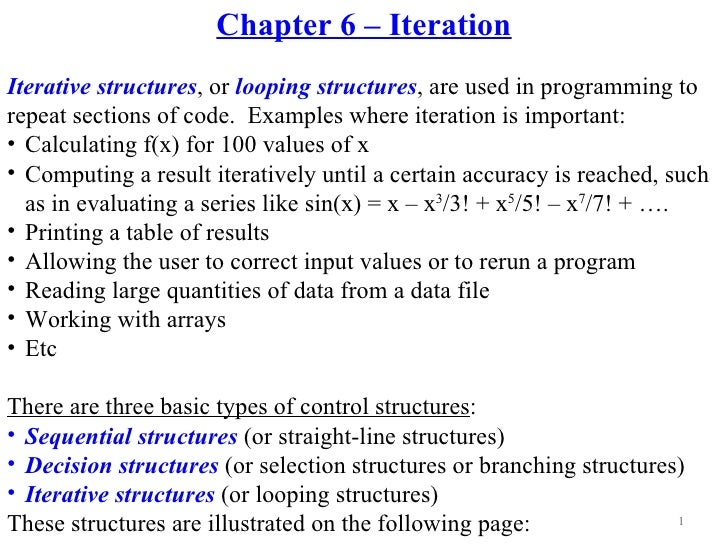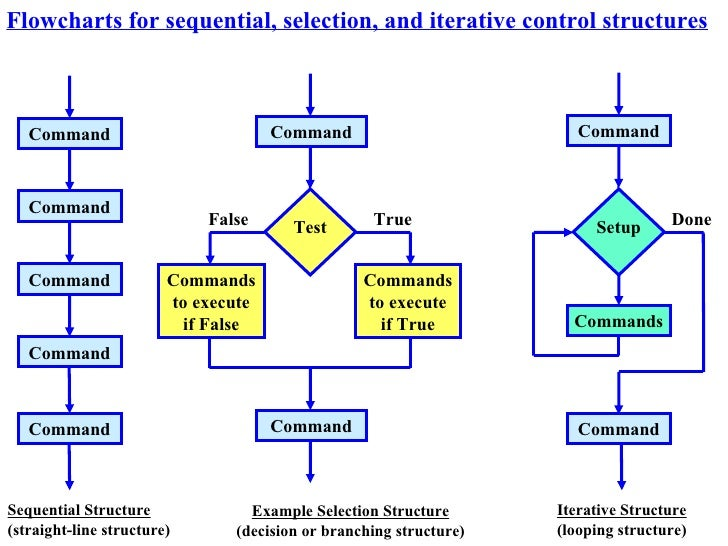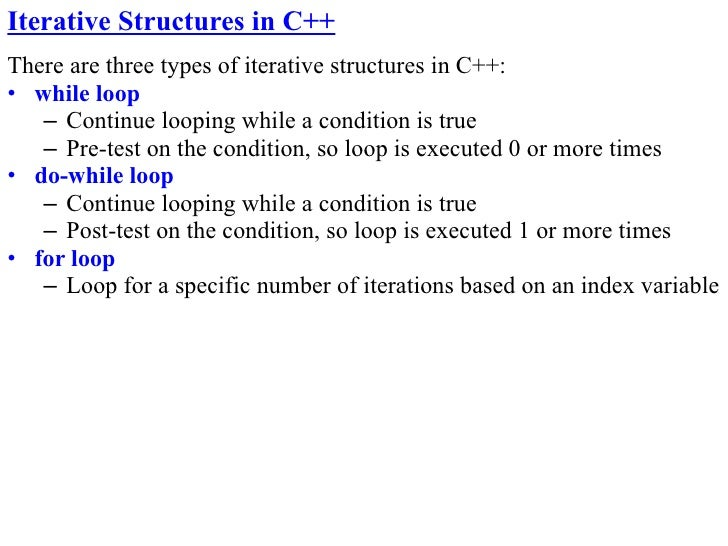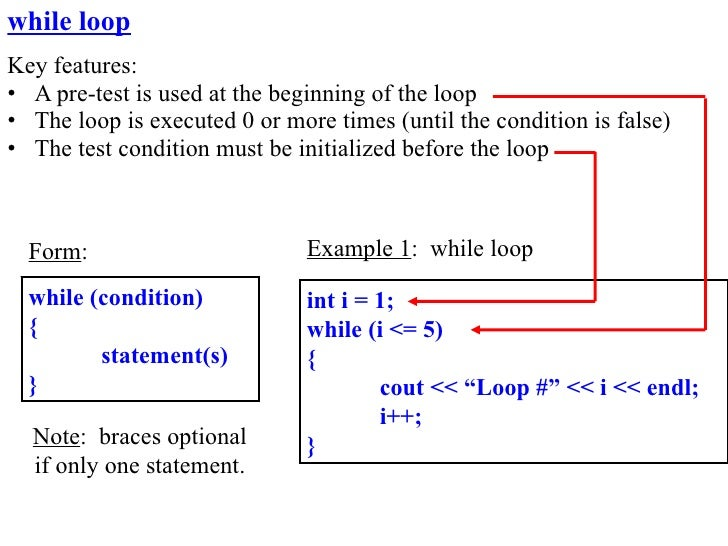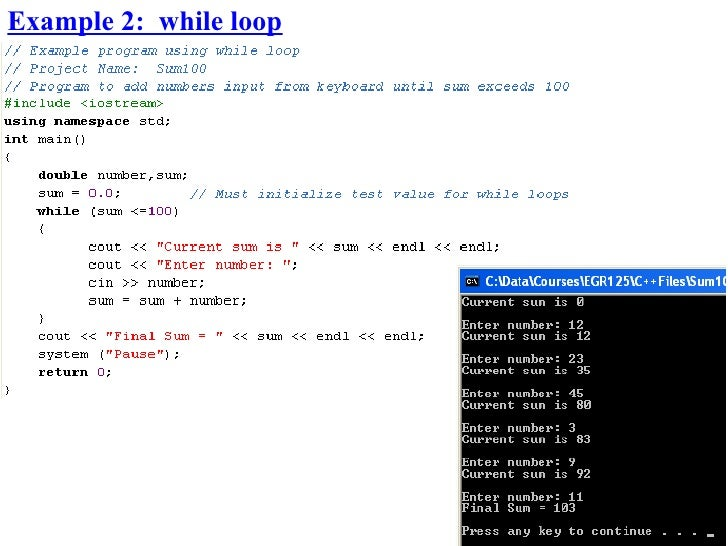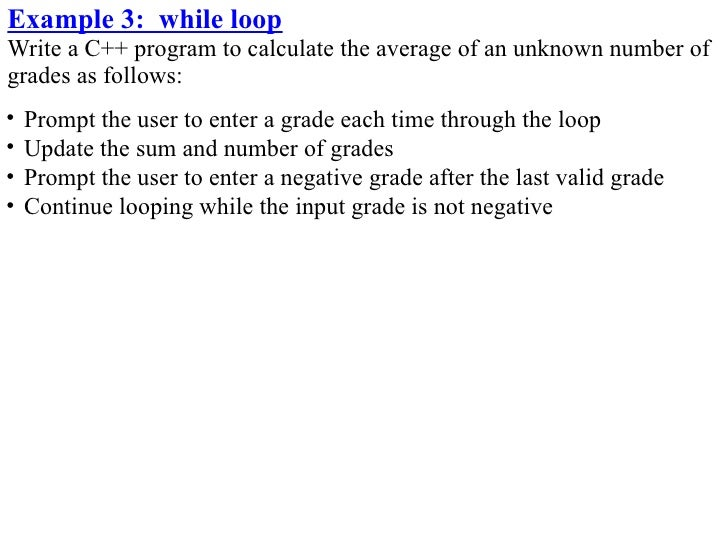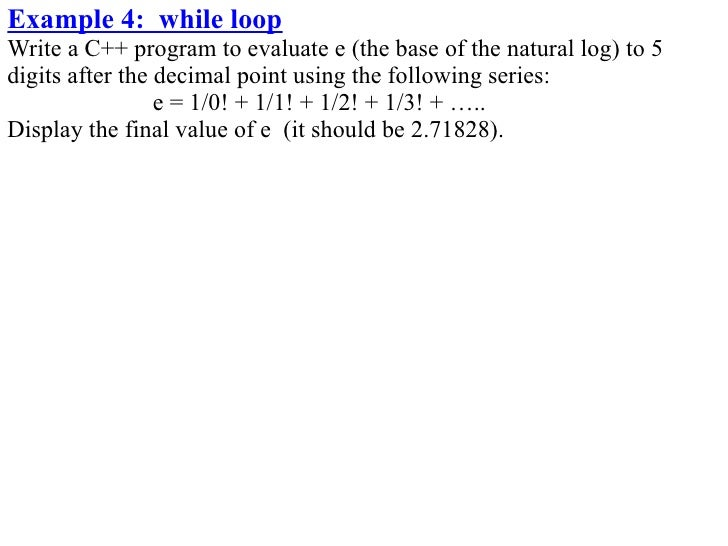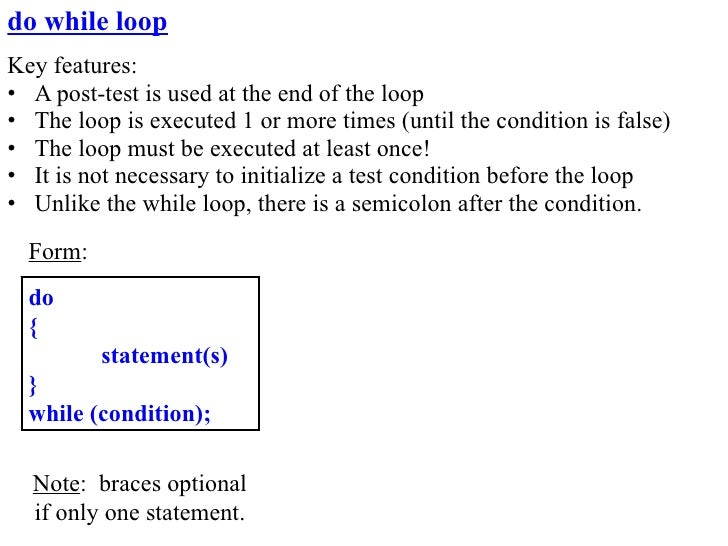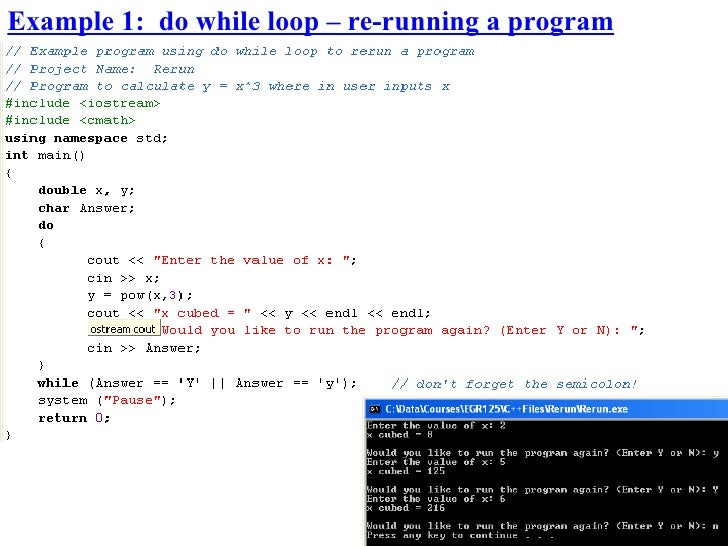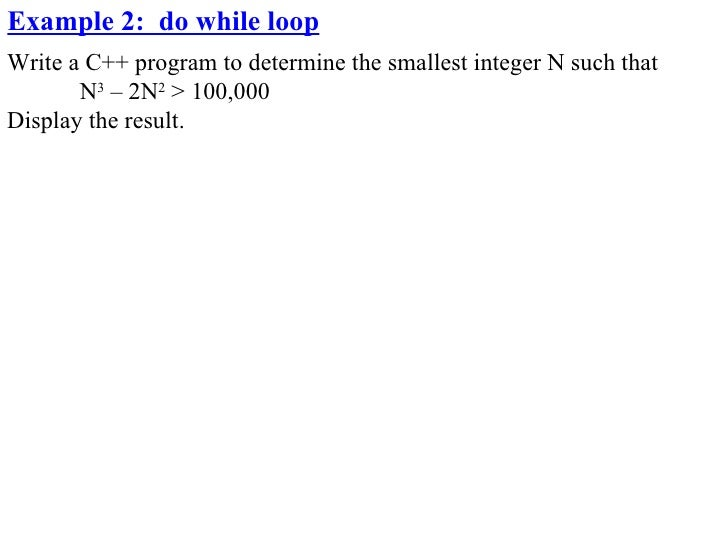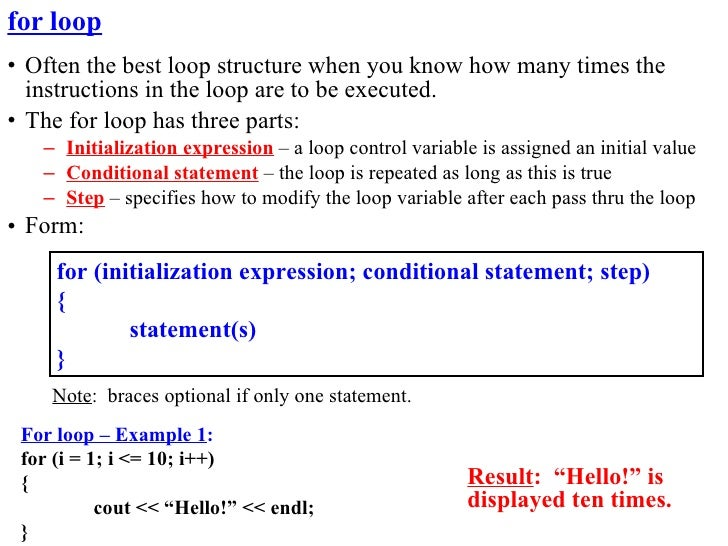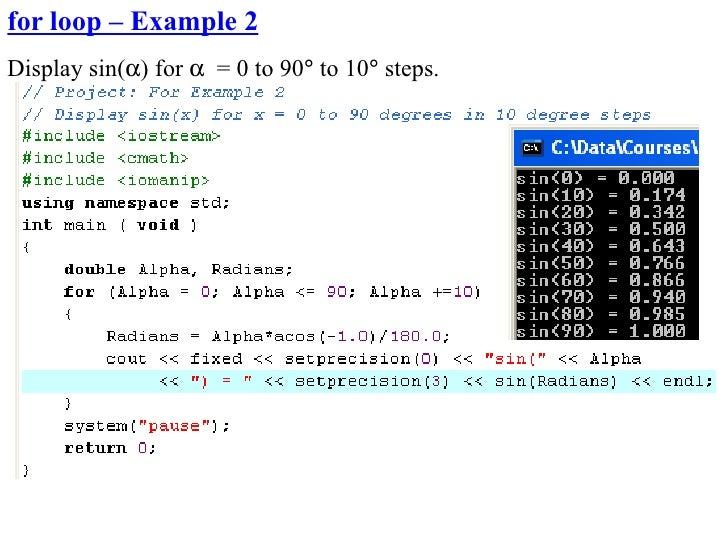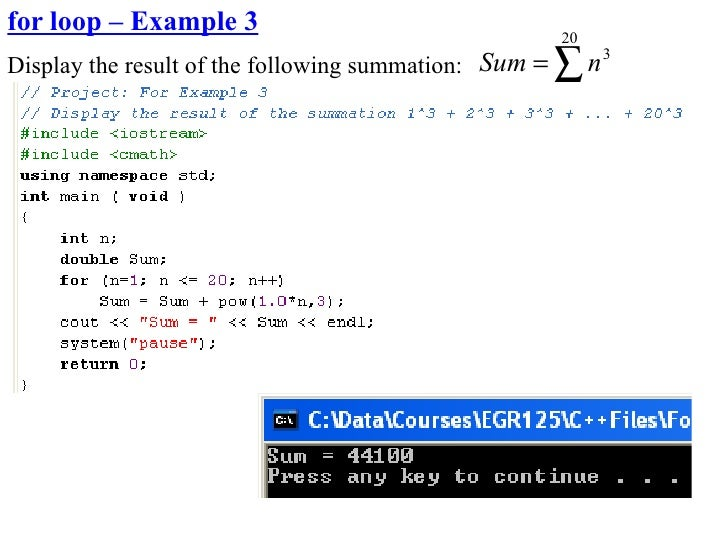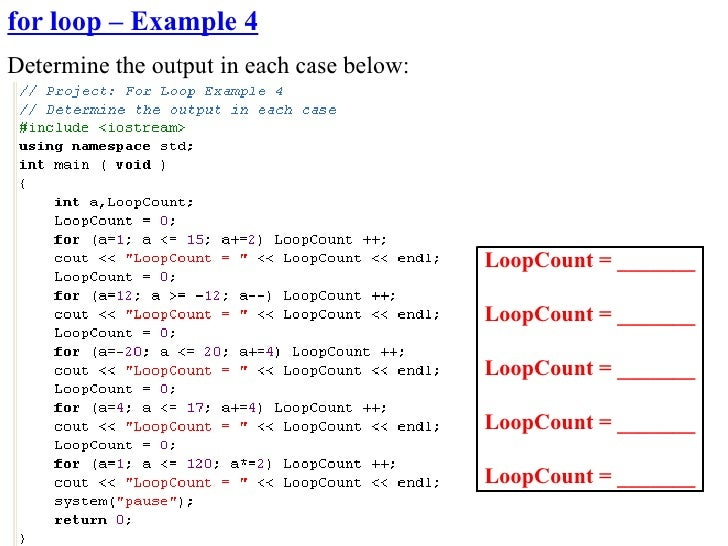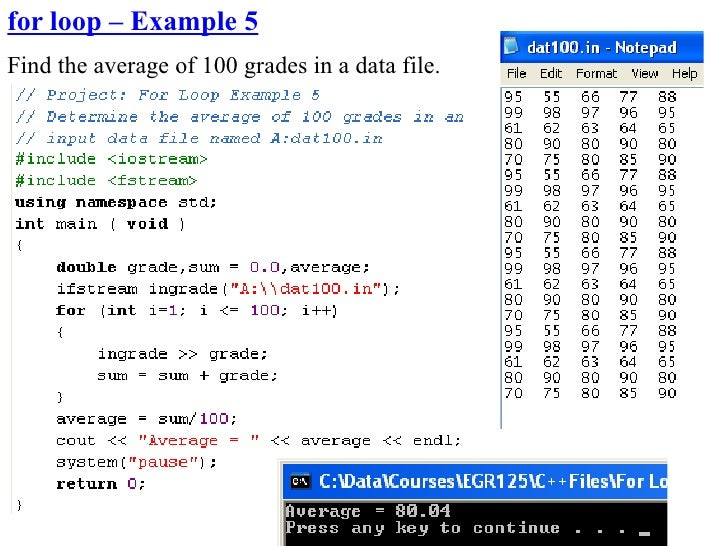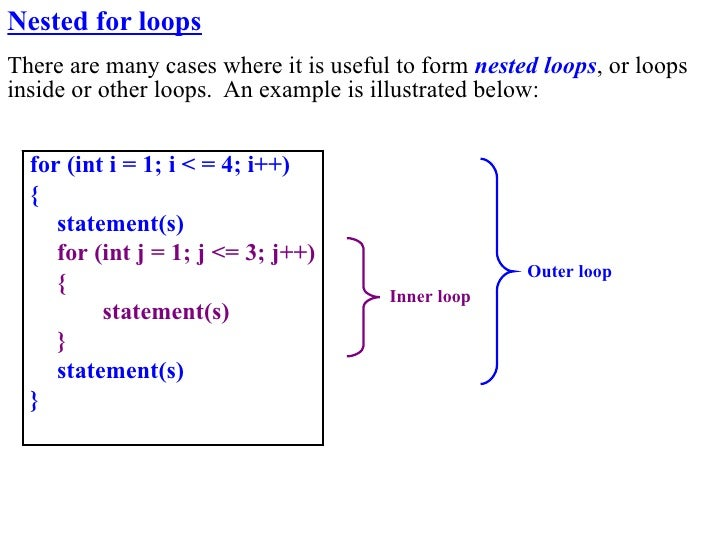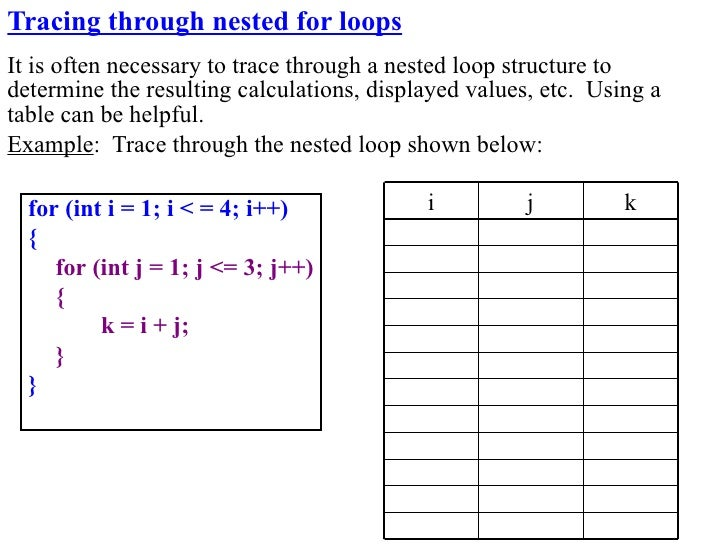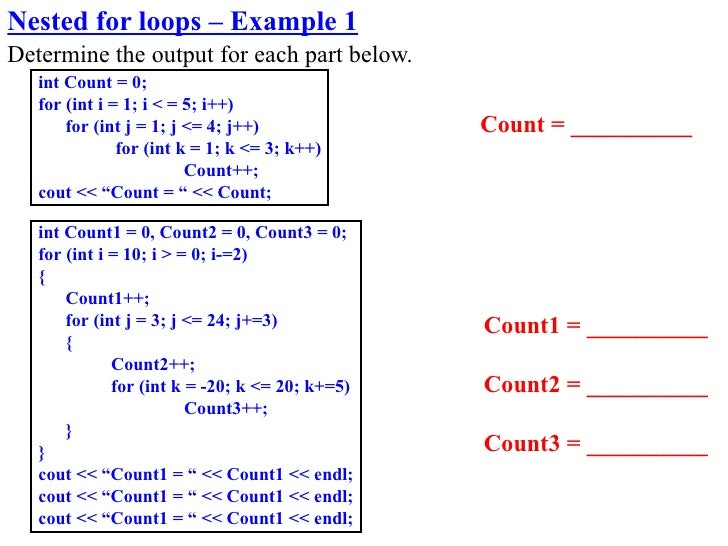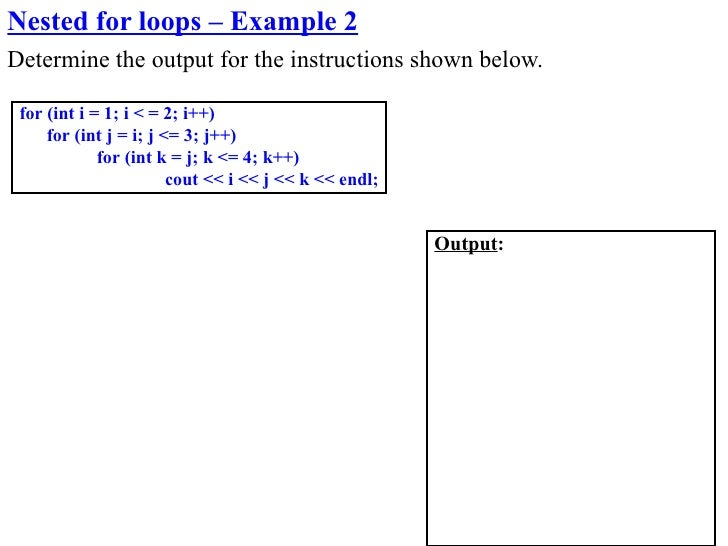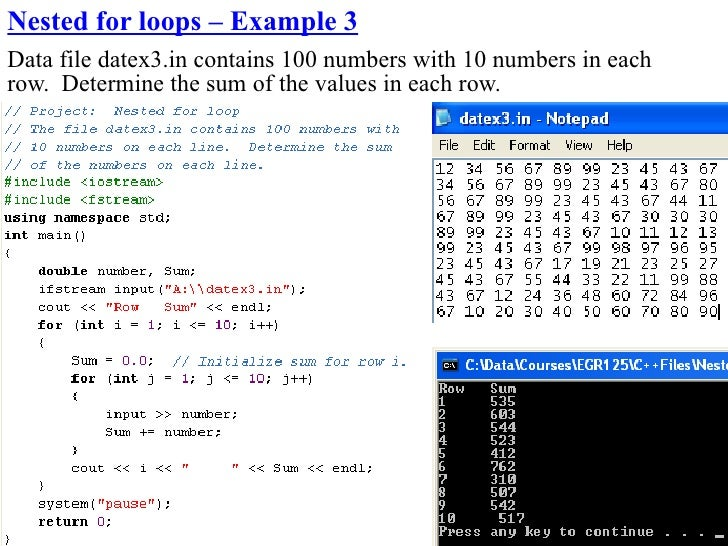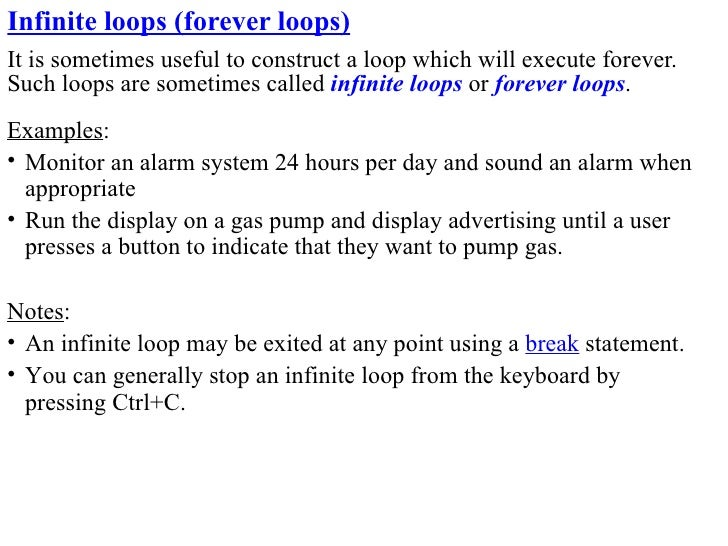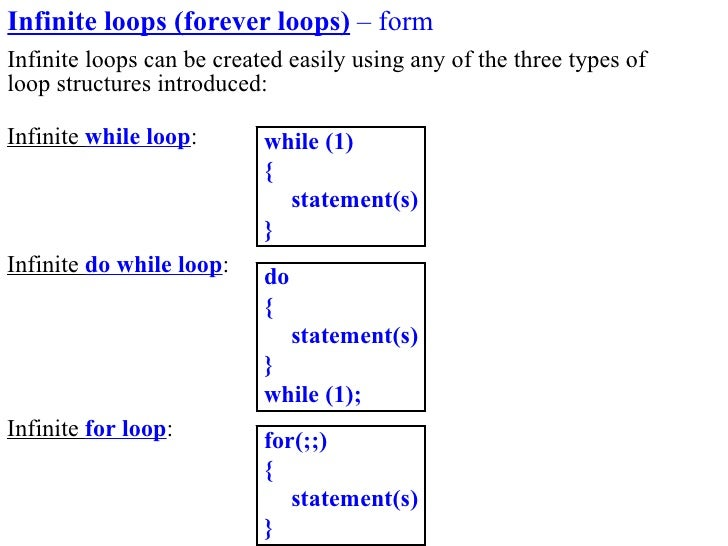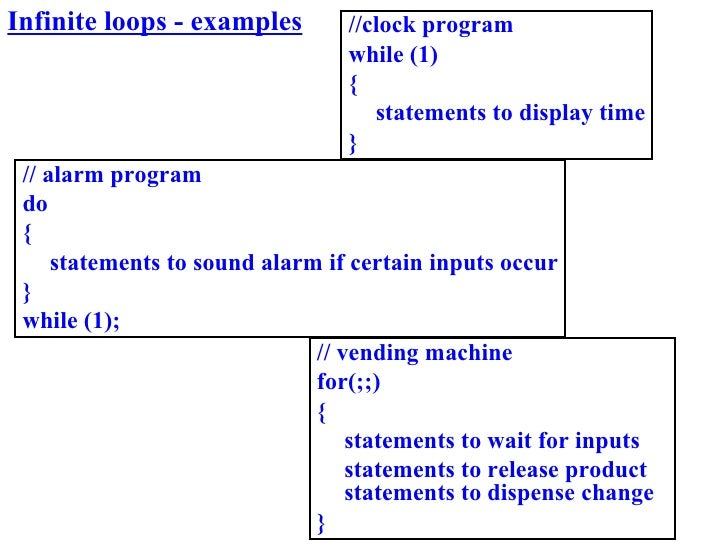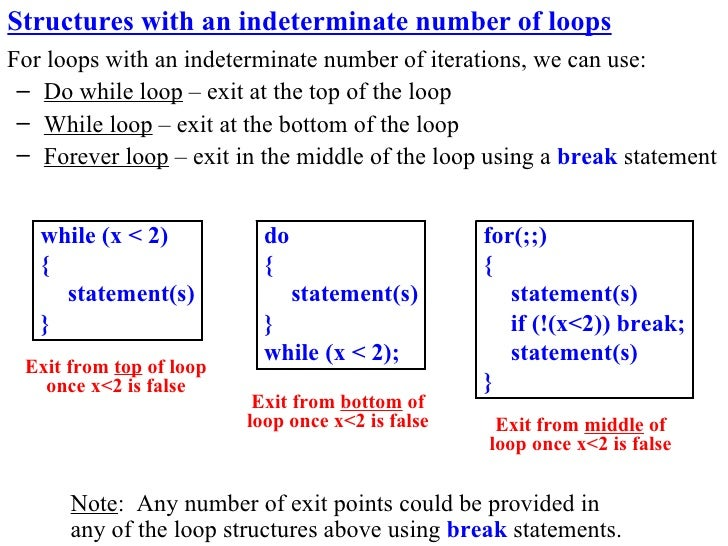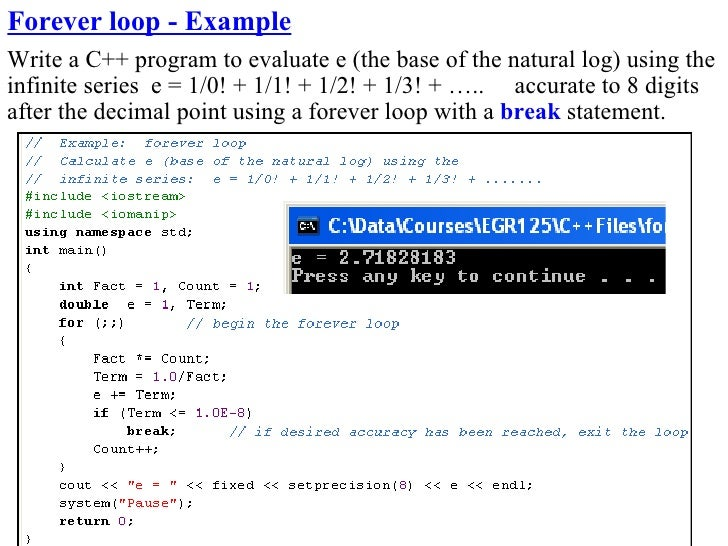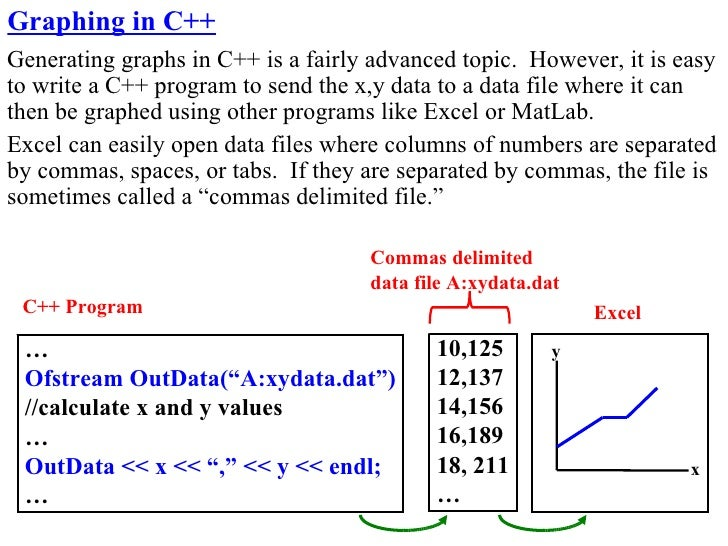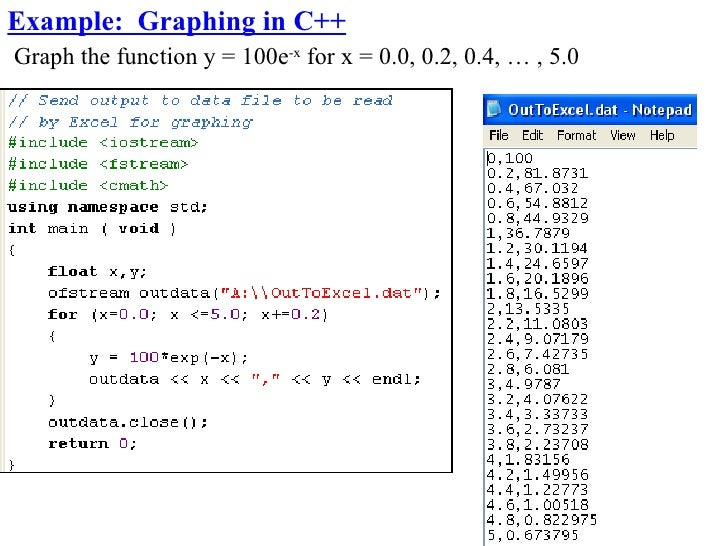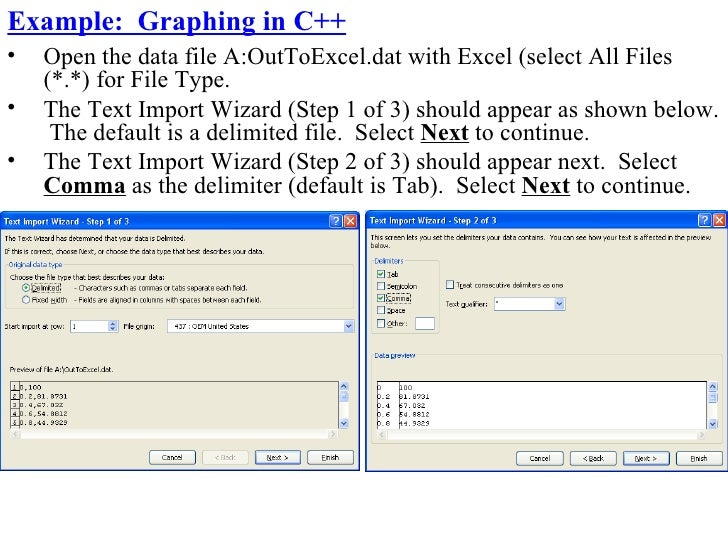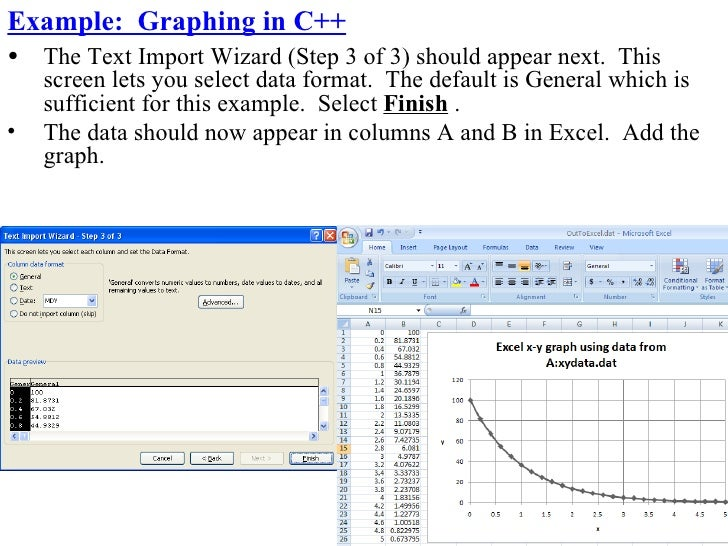Upcoming SlideShare
×
• Full Name
Comment goes here.

Are you sure you want to Yes No• Be the first to comment

### Iteration

1. 1. Chapter 6 – Iteration Iterative structures, or looping structures, are used in programming to repeat sections of code. Examples where iteration is important: • Calculating f(x) for 100 values of x • Computing a result iteratively until a certain accuracy is reached, such as in evaluating a series like sin(x) = x – x3/3! + x5/5! – x7/7! + …. • Printing a table of results • Allowing the user to correct input values or to rerun a program • Reading large quantities of data from a data file • Working with arrays • Etc There are three basic types of control structures: • Sequential structures (or straight-line structures) • Decision structures (or selection structures or branching structures) • Iterative structures (or looping structures) These structures are illustrated on the following page: 1
2. 2. Flowcharts for sequential, selection, and iterative control structures Command Command Command Command False Test True Setup Done Command Commands Commands to execute to execute if False if True Commands Command Command Command Command Sequential Structure Example Selection Structure Iterative Structure 2 (straight-line structure) (decision or branching structure) (looping structure)
3. 3. Iterative Structures in C++ There are three types of iterative structures in C++: • while loop – Continue looping while a condition is true – Pre-test on the condition, so loop is executed 0 or more times • do-while loop – Continue looping while a condition is true – Post-test on the condition, so loop is executed 1 or more times • for loop – Loop for a specific number of iterations based on an index variable
4. 4. while loop Key features: • A pre-test is used at the beginning of the loop • The loop is executed 0 or more times (until the condition is false) • The test condition must be initialized before the loop Form: Example 1: while loop while (condition) int i = 1; { while (i <= 5) statement(s) { } cout << “Loop #” << i << endl; i++; Note: braces optional } if only one statement.
5. 5. Example 2: while loop
6. 6. Example 3: while loop Write a C++ program to calculate the average of an unknown number of grades as follows: • Prompt the user to enter a grade each time through the loop • Update the sum and number of grades • Prompt the user to enter a negative grade after the last valid grade • Continue looping while the input grade is not negative
7. 7. Example 4: while loop Write a C++ program to evaluate e (the base of the natural log) to 5 digits after the decimal point using the following series: e = 1/0! + 1/1! + 1/2! + 1/3! + ….. Display the final value of e (it should be 2.71828).
8. 8. do while loop Key features: • A post-test is used at the end of the loop • The loop is executed 1 or more times (until the condition is false) • The loop must be executed at least once! • It is not necessary to initialize a test condition before the loop • Unlike the while loop, there is a semicolon after the condition. Form: do { statement(s) } while (condition); Note: braces optional if only one statement.
9. 9. Example 1: do while loop – re-running a program
10. 10. Example 2: do while loop Write a C++ program to determine the smallest integer N such that N3 – 2N2 > 100,000 Display the result.
11. 11. for loop • Often the best loop structure when you know how many times the instructions in the loop are to be executed. • The for loop has three parts: – Initialization expression – a loop control variable is assigned an initial value – Conditional statement – the loop is repeated as long as this is true – Step – specifies how to modify the loop variable after each pass thru the loop • Form: for (initialization expression; conditional statement; step) { statement(s) } Note: braces optional if only one statement. For loop – Example 1: for (i = 1; i <= 10; i++) { Result: “Hello!” is cout << “Hello!” << endl; displayed ten times. }
12. 12. for loop – Example 2 Display sin(α) for α = 0 to 90° to 10° steps.
13. 13. for loop – Example 3 20 Display the result of the following summation: Sum = ∑ n 3 n =1
14. 14. for loop – Example 4 Determine the output in each case below: LoopCount = _______ LoopCount = _______ LoopCount = _______ LoopCount = _______ LoopCount = _______
15. 15. for loop – Example 5 Find the average of 100 grades in a data file.
16. 16. Nested for loops There are many cases where it is useful to form nested loops, or loops inside or other loops. An example is illustrated below: for (int i = 1; i < = 4; i++) { statement(s) for (int j = 1; j <= 3; j++) Outer loop { Inner loop statement(s) } statement(s) }
17. 17. Tracing through nested for loops It is often necessary to trace through a nested loop structure to determine the resulting calculations, displayed values, etc. Using a table can be helpful. Example: Trace through the nested loop shown below: for (int i = 1; i < = 4; i++) i j k { for (int j = 1; j <= 3; j++) { k = i + j; } }
18. 18. Nested for loops – Example 1 Determine the output for each part below. int Count = 0; for (int i = 1; i < = 5; i++) for (int j = 1; j <= 4; j++) Count = __________ for (int k = 1; k <= 3; k++) Count++; cout << “Count = “ << Count; int Count1 = 0, Count2 = 0, Count3 = 0; for (int i = 10; i > = 0; i-=2) { Count1++; for (int j = 3; j <= 24; j+=3) Count1 = __________ { Count2++; for (int k = -20; k <= 20; k+=5) Count2 = __________ Count3++; } } Count3 = __________ cout << “Count1 = “ << Count1 << endl; cout << “Count1 = “ << Count1 << endl; cout << “Count1 = “ << Count1 << endl;
19. 19. Nested for loops – Example 2 Determine the output for the instructions shown below. for (int i = 1; i < = 2; i++) for (int j = i; j <= 3; j++) for (int k = j; k <= 4; k++) cout << i << j << k << endl; Output:
20. 20. Nested for loops – Example 3 Data file datex3.in contains 100 numbers with 10 numbers in each row. Determine the sum of the values in each row.
21. 21. Infinite loops (forever loops) It is sometimes useful to construct a loop which will execute forever. Such loops are sometimes called infinite loops or forever loops. Examples: • Monitor an alarm system 24 hours per day and sound an alarm when appropriate • Run the display on a gas pump and display advertising until a user presses a button to indicate that they want to pump gas. Notes: • An infinite loop may be exited at any point using a break statement. • You can generally stop an infinite loop from the keyboard by pressing Ctrl+C.
22. 22. Infinite loops (forever loops) – form Infinite loops can be created easily using any of the three types of loop structures introduced: Infinite while loop: while (1) { statement(s) } Infinite do while loop: do { statement(s) } while (1); Infinite for loop: for(;;) { statement(s) }
23. 23. Infinite loops - examples //clock program while (1) { statements to display time } // alarm program do { statements to sound alarm if certain inputs occur } while (1); // vending machine for(;;) { statements to wait for inputs statements to release product statements to dispense change }
24. 24. Structures with an indeterminate number of loops For loops with an indeterminate number of iterations, we can use: – Do while loop – exit at the top of the loop – While loop – exit at the bottom of the loop – Forever loop – exit in the middle of the loop using a break statement while (x < 2) do for(;;) { { { statement(s) statement(s) statement(s) } } if (!(x<2)) break; while (x < 2); statement(s) Exit from top of loop once x<2 is false } Exit from bottom of loop once x<2 is false Exit from middle of loop once x<2 is false Note: Any number of exit points could be provided in any of the loop structures above using break statements.
25. 25. Forever loop - Example Write a C++ program to evaluate e (the base of the natural log) using the infinite series e = 1/0! + 1/1! + 1/2! + 1/3! + ….. accurate to 8 digits after the decimal point using a forever loop with a break statement.
26. 26. Graphing in C++ Generating graphs in C++ is a fairly advanced topic. However, it is easy to write a C++ program to send the x,y data to a data file where it can then be graphed using other programs like Excel or MatLab. Excel can easily open data files where columns of numbers are separated by commas, spaces, or tabs. If they are separated by commas, the file is sometimes called a “commas delimited file.” Commas delimited data file A:xydata.dat C++ Program Excel … 10,125 y Ofstream OutData(“A:xydata.dat”) 12,137 //calculate x and y values 14,156 … 16,189 OutData << x << “,” << y << endl; 18, 211 x … …
27. 27. Example: Graphing in C++ Graph the function y = 100e-x for x = 0.0, 0.2, 0.4, … , 5.0
28. 28. Example: Graphing in C++ • Open the data file A:OutToExcel.dat with Excel (select All Files (*.*) for File Type. • The Text Import Wizard (Step 1 of 3) should appear as shown below. The default is a delimited file. Select Next to continue. • The Text Import Wizard (Step 2 of 3) should appear next. Select Comma as the delimiter (default is Tab). Select Next to continue.
29. 29. Example: Graphing in C++ • The Text Import Wizard (Step 3 of 3) should appear next. This screen lets you select data format. The default is General which is sufficient for this example. Select Finish . • The data should now appear in columns A and B in Excel. Add the graph.

### Be the first to comment

•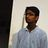#### amanullashaik3304

Apr. 24, 2014
•#### HARSHALBORDIYA

Sep. 13, 2020

Total views

20,056

On Slideshare

0

From embeds

0

Number of embeds

27

306

Shares

0